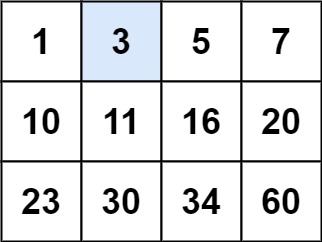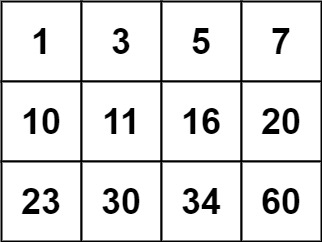# Search a 2D Matrix

## 1: U-nderstand

Understand what the interviewer is asking for by using test cases and questions about the problem.

• Established a set (2-3) of test cases to verify their own solution later.
• Established a set (1-2) of edge cases to verify their solution handles complexities.
• Have fully understood the problem and have no clarifying questions.
• Have you verified any Time/Space Constraints for this problem?
• Can the input matrix be blank?
• Let’s assume the matrix is not blank. We don’t need to consider empty inputs.
• Can the row size be different from the column size?
• Yes, the row size can be different from the column size
• Is the target always in the matrix?
• No, the target might not be in the matrix
• What is the time and space constraints?
• Time complexity should be `O(log(M*N))`, `M` being the rows of the matrix and `N` being the columns of matrix. Space complexity should be `O(1)`, excluding the recursive stack.
``````HAPPY CASE
Input: matrix = [[1,3,5,7],[10,11,16,20],[23,30,34,60]], target = 3
Output: true````````````Input: matrix = [[1,3,5,7],[10,11,16,20],[23,30,34,60]], target = 13
Output: false````````````EDGE CASE
Input: board = [], target = 1
Output: true
``````

## 2: M-atch

Match what this problem looks like to known categories of problems, e.g. Linked List or Dynamic Programming, and strategies or patterns in those categories.

For 2D-Array, common solution patterns include:

• Perform a BFS/DFS Search through the 2D Array
• A search through the 2D Array (either BFS or DFS) can help us, but at the cost of n*m.
• Hash the 2D Array in some way to help with the Strings
• Hashing would not help us acheive the runtime O(log(n*m))
• Create/Utilize a Trie
• A Trie would not help us much in this problem since we are not trying to determine anything about a sequence of characters.

## 3: P-lan

Plan the solution with appropriate visualizations and pseudocode.

General Idea: Let's perform binary search with both the columns and the rows

``````1) Perform binary search with each row
2) Perform early exit if target is out of range
3) Perform binary search with each number in identified row and return True if found.

⚠️ Common Mistakes

• log(m*n) runtime can only mean one thing, binary search

## 4: I-mplement

Implement the code to solve the algorithm.

``````class Solution:
def searchMatrix(self, matrix: List[List[int]], target: int) -> bool:
# Perform binary search with each row
top, bottom = 0, len(matrix) - 1
while top <= bottom:
mid = (top + bottom) // 2

if target < matrix[mid]:
bottom = mid - 1
elif target > matrix[mid][-1]:
top = mid + 1
else:
break

# Perform early exit if target is out of range
if top > bottom:
return False

# Perform binary search with each number in identified row and return True if found.
left, right = 0, len(matrix[mid]) - 1
row = matrix[mid]
while left <= right:
mid = (left + right) // 2
if row[mid] == target:
return True
elif row[mid] < target:
left = mid + 1
else:
right = mid - 1

return False``````
``````class Solution {
public boolean searchMatrix(int[][] matrix, int target) {
// Perform binary search with each row
int r1 = 0;
int r2 = matrix.length - 1;
int c1 = 0;
int c2 = matrix.length - 1;
int row = 0;
while (r1 <= r2) {
int mid = (r1 + r2) / 2;
if (target < matrix[mid][c1]) {
r2 = mid - 1;
}
else if (target > matrix[mid][c2]) {
r1 = mid + 1;
}
else {
row = mid;
break;
}
}

if (r1 > r2) {
return false;
}

// Perform binary search with each number in identified row and return True if found.
while (c1 <= c2) {
int mid = (c1 + c2) / 2;
if (matrix[row][mid] == target) {
return true;
}
else if (target < matrix[row][mid]) {
c2 = mid - 1;
}
else {
c1 = mid + 1;
}
}

return false;
}
}``````

## 5: R-eview

Review the code by running specific example(s) and recording values (watchlist) of your code's variables along the way.

• Trace through your code with an input to check for the expected output
• Catch possible edge cases and off-by-one errors

## 6: E-valuate

Evaluate the performance of your algorithm and state any strong/weak or future potential work.

Assume `N` represents the number of rows in 2D-array. Assume `M` represents the number of columns in 2D-array.

• Time Complexity: `O(log(N * M))` we used binary search on each row, then on each column of that row in the 2D-Array
• Space Complexity: `O(1)`, we only need to store a few variables.